# In the second test, you will prepare 10 mL of each solution containing 0.1%, 0.2%, 0.5%, 0.8%, 1%, 5%, and 10% H2O2. Explain how this will be done using a 10% stock solution of H2O2 to prepare the 5 % solution and a stock solution of 1 % H2O2 to prepare the remaining

Question
45 views

In the second test, you will prepare 10 mL of each solution containing 0.1%, 0.2%, 0.5%, 0.8%, 1%, 5%, and 10% H2O2. Explain how this will be done using a 10% stock solution of H2O2 to prepare the 5 % solution and a stock solution of 1 % H2O2 to prepare the remaining

check_circle

Step 1

Amount needed for the preparation of 5 mL, 10% stock solution of H2O2 from the 10% stock solution can be calculated as follows: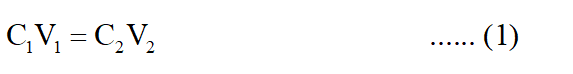Where C1 and V1 are the concentration and volume of initial solution and C2 and V2 are the concentration and volume of final solution.

Putting the values in equation (1)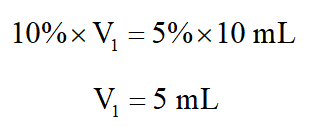Thus 5 mL of 10% stock solution of H2O2 is needed to prepare 5% of 10 mL stock solution.

Step 2

Amount of  H2O2 needed for the preparation of 10 mL of 0.1% stock solution from 1% stock solution can be calculated as follows: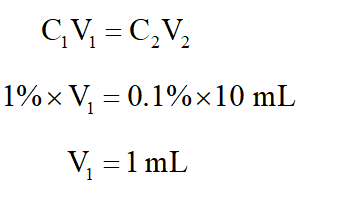Amount of  H2O2 needed for the preparation of 10 mL of 0.2% stock solution from 1% stock solution can be calculated as follows: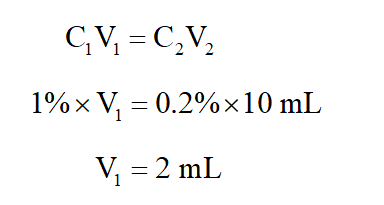Amount of  H2O2 needed for the preparation of 10 mL of 0.5% stock solution from 1% stock solution can be calculated as follows: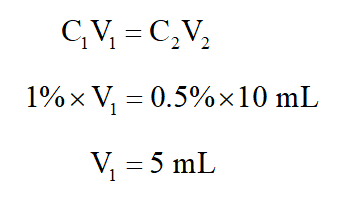...

### Want to see the full answer?

See Solution

#### Want to see this answer and more?

Solutions are written by subject experts who are available 24/7. Questions are typically answered within 1 hour.*

See Solution
*Response times may vary by subject and question.
Tagged in